# Factoring with FOIL, Graphing Parabolas and Solving Quadratics: Tutoring Solution Chapter Exam

Exam Instructions:

Choose your answers to the questions and click 'Next' to see the next set of questions. You can skip questions if you would like and come back to them later with the yellow "Go To First Skipped Question" button. When you have completed the practice exam, a green submit button will appear. Click it to see your results. Good luck!

Answered 0 of 30

### Page 1

#### Question 1 1. Solve the equation.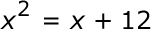#### Question 3 3. Rewrite the quadratic below in standard form: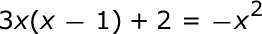#### Question 5 5. Find the roots of the equation.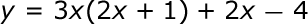### Page 2

#### Question 7 7. Expressing the equation below in the vertex form will give a value of 'h' = _____ .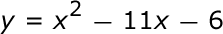#### Question 10 10. If the equation below told you a ball was 'h' feet in the air after 't' seconds, what would be the first step to finding out when it hit the ground?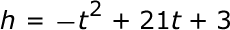### Page 3

#### Question 15 15. Find the value for c and rewrite the equation in the vertex form.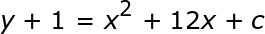### Page 4

#### Question 18 18. What is the b-value of the quadratic equation below?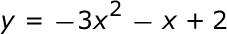#### Question 19 19. Which of the following are the solutions to the equation below?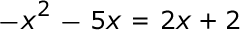### Page 5

#### Question 23 23. When completing the square on the quadratic equation listed below, how is c calculated?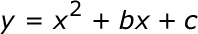#### Question 24 24. Solve the equation below.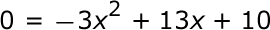### Page 6

#### Question 26 26. Find the roots of the equation below.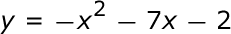#### Question 27 27. What would the first step be in completing the square of the following equation?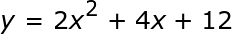#### Question 28 28. Rewrite the following equation in the vertex form.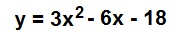#### Factoring with FOIL, Graphing Parabolas and Solving Quadratics: Tutoring Solution Chapter Exam Instructions

Choose your answers to the questions and click 'Next' to see the next set of questions. You can skip questions if you would like and come back to them later with the yellow "Go To First Skipped Question" button. When you have completed the practice exam, a green submit button will appear. Click it to see your results. Good luck!

Support